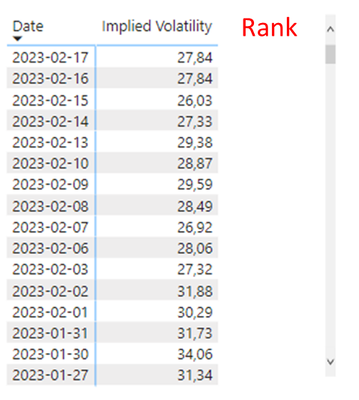cancel
Showing results for
Did you mean:Frequent Visitor

## RANKX for Rolling last 12 Month

Hi all,

I have some daily Data ('Implied Volatility', but only for working days) and i'm struggling to build a measure that gives me a rolling-1-year-Rank measure. If i dont select any Date(s) for row (e.g. in a card), it should show me the current Rank of last 1 year from today on.

Probably it is something like...

Rank = RANKX (

CALCULATETABLE(...),

[newColumnImpliedVolatility]

)

``Implied Volatility Measure = CALCULATE(AVERAGE(Fact_UnderlyingDaily[ImpliedVolatility]))``1 ACCEPTED SOLUTIONCommunity Champion

try like:

Ranking =
VAR _date = MAX(TableName[Date])
RETURN
RANKX(
CALCULATETABLE(
ALLSELECTED(TableName[Date]),
TableName[Date]<=_date,
TableName[Date]>=EDATE(_date, -12)
),
[Implied Volatility]
)
2 REPLIES 2Frequent Visitor

Hi @FreemanZ  thank you for your solution.

One Question: why do we need ALLSELECTED?Community Champion

try like:

Ranking =
VAR _date = MAX(TableName[Date])
RETURN
RANKX(
CALCULATETABLE(
ALLSELECTED(TableName[Date]),
TableName[Date]<=_date,
TableName[Date]>=EDATE(_date, -12)
),
[Implied Volatility]
)